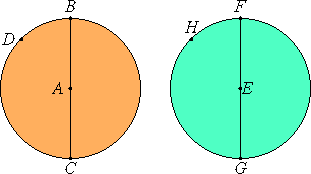# Definition 1

Equal circles are those whose diameters are equal, or whose radii are equal.

## Guide

This should not be a definition but a postulate or a theorem. The subject of the area of circles is developed in Proposition XII.2 and this definition is not used there. Instead the concept of equality, or rather, inequality is the same as it is in the rest of the Elements. For instance, if one figure is the contained in another, then the first is less than the other. Thus, there is a prior definition for the equality of two figures.Two circles are illustrated, namely circle BCD and circle FGH. The center of circle BCD is A, while the center of circle FGH is E. They are equal by Euclid’s definition since their diameters BC and FG are equal, or since their radii AB and EF are equal.